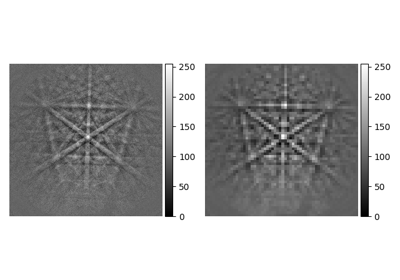# rebin#

EBSD.rebin(*args, **kwargs)[source]#

Rebin the signal into a smaller or larger shape, based on linear interpolation. Specify either new_shape or scale. Scale of 1 means no binning and scale less than one results in up-sampling.

This docstring was copied from HyperSpy’s Signal2D.rebin. Some inconsistencies with the kikuchipy version may exist.

Parameters:
new_shape`list` (`of` `floats` or `integer`) `or` `None`

For each dimension specify the new_shape. This will internally be converted into a `scale` parameter.

scale`list` (`of` `floats` or `integer`) `or` `None`

For each dimension, specify the new:old pixel ratio, e.g. a ratio of 1 is no binning and a ratio of 2 means that each pixel in the new spectrum is twice the size of the pixels in the old spectrum. The length of the list should match the dimension of the Signal’s underlying data array. Note : Only one of `scale` or `new_shape` should be specified, otherwise the function will not run

cropbool

Whether or not to crop the resulting rebinned data (default is `True`). When binning by a non-integer number of pixels it is likely that the final row in each dimension will contain fewer than the full quota to fill one pixel. For example, a 5*5 array binned by 2.1 will produce two rows containing 2.1 pixels and one row containing only 0.8 pixels. Selection of `crop=True` or `crop=False` determines whether or not this “black” line is cropped from the final binned array or not. Please note that if ``crop=False`` is used, the final row in each dimension may appear black if a fractional number of pixels are left over. It can be removed but has been left to preserve total counts before and after binning.

dtype{`None`, `numpy.dtype`, “same”}

Specify the dtype of the output. If None, the dtype will be determined by the behaviour of `numpy.sum()`, if “same”, the dtype will be kept the same. Default is None.

out`BaseSignal` (`or` `subclasses`) `or` `None`

If `None`, a new Signal is created with the result of the operation and returned (default). If a Signal is passed, it is used to receive the output of the operation, and nothing is returned.

Returns:
s`BaseSignal` (`or` `subclass`)

The resulting cropped signal.

Raises:
`NotImplementedError`

If trying to rebin over a non-uniform axis.

Examples

```>>> spectrum = hs.signals.EDSTEMSpectrum(np.ones([4, 4, 10]))
>>> spectrum.data[1, 2, 9] = 5
>>> print(spectrum)
<EDXTEMSpectrum, title: dimensions: (4, 4|10)>
>>> print ('Sum = ', sum(sum(sum(spectrum.data))))
Sum = 164.0
```
```>>> scale = [2, 2, 5]
>>> test = spectrum.rebin(scale)
>>> print(test)
<EDSTEMSpectrum, title: dimensions (2, 2|2)>
>>> print('Sum = ', sum(sum(sum(test.data))))
Sum =  164.0
```
```>>> s = hs.signals.Signal1D(np.ones((2, 5, 10), dtype=np.uint8)
>>> print(s)
<Signal1D, title: , dimensions: (5, 2|10)>
>>> print(s.data.dtype)
uint8
```

Use dtype=np.unit16 to specify a dtype

```>>> s2 = s.rebin(scale=(5, 2, 1), dtype=np.uint16)
>>> print(s2.data.dtype)
uint16
```

Use dtype=”same” to keep the same dtype

```>>> s3 = s.rebin(scale=(5, 2, 1), dtype="same")
>>> print(s3.data.dtype)
uint8
```

By default dtype=None, the dtype is determined by the behaviour of numpy.sum, in this case, unsigned integer of the same precision as the platform interger

```>>> s4 = s.rebin(scale=(5, 2, 1))
>>> print(s4.data.dtype)
uint64
```

## Examples using `EBSD.rebin`#Pattern binning

Pattern binning